# Math

Here is an order form listing all of our math materials.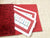Addition Equations - Color-coded Numerals: Laminated - mathladd \$13.95 USDAddition Equations - Black Numerals: Unlaminated - mathnadd-bl \$2.95 USDAddition Equations - Black Numerals: Laminated - mathladd-bl \$4.50 USD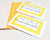Multiplication Equations - Color-coded Numerals: Laminated - mathlmult \$13.95 USDMultiplication Equations - Black Numerals: Unlaminated - mathnmult-bl \$2.95 USDMultiplication Equations - Black Numerals: Laminated - mathlmult-bl \$4.50 USD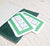Subtraction Equations - Color-coded Numerals: Laminated - mathlsubt \$13.95 USDSubtraction Equations - Black Numerals: Laminated - mathlsubt-bl \$4.50 USD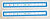Short Division Equations - Color-coded Numerals: Laminated - mathlsdiv \$13.95 USDShort Division Equations - Black Numerals: Unlaminated - mathnsdiv-bl \$2.95 USDShort Division Equations - Black Numerals: Laminated - mathlsdiv-bl \$4.50 USD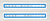Long Division Equations - Color-coded Numerals: Unlaminated - mathnldiv \$4.50 USDLong Division Equations - Color-coded Numerals: Laminated - mathlldiv \$13.95 USD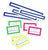Math Set - Color-coded Numerals: Laminated - mathlset \$59.95 USDMath Set - Cloth Pouch Set/5 - pmathset \$36.75 USD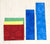Math Cloth Pouches - Math Set/5 - p1mathset/5 \$43.95 USDMath Cloth Pouches - Addition - p1mred \$8.95 USDMath Cloth Pouches - Multiplication - p1mlem \$8.95 USDMath Cloth Pouches - Subtraction - p1mgreen \$8.95 USDMath Cloth Pouches - Short Division - p1sdiv \$8.95 USDMath Cloth Pouches - Long Division - p1ldiv \$8.95 USDImperfect Addition Equations - dismathladd \$6.75 USDImperfect Subtraction Equations - dismathlsubt \$6.75 USDImperfect Long Division Equations - Color-coded Numerals: Laminated - dismathlldiv \$6.75 USD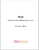Math Album (EC) - View-Only Digital Math Album: Instructor Edition - albmath-tr-ggl \$49.95 USDMath Album (EC) - View-Only Digital Math Album: Student Edition - albmath-st-ggl \$29.95 USDMath Album (EC) - Printed Math Album: Instructor Edition - albmath-tr \$199.95 USDMath Album (EC) - Printed Math Album: Student Edition - albmath-st \$99.95 USDMath Album (EC) - Printable Digital Math Album: Instructor Edition - albmath-tr-pdc \$79.95 USDMath Album (EC) - Printable Digital Math Album: Student Edition - albmath-st-pdc \$44.95 USD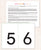Sandpaper Numbers Digital - sp#-pdc \$2.95 USD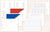Addition Strip Board Digital - adbrds-pdc \$4.95 USD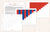Number Rods/Red Rods Digital - numrods-pdc \$2.95 USD